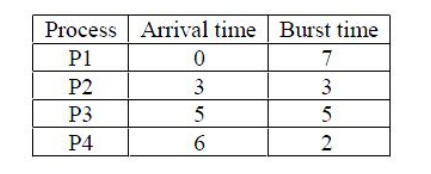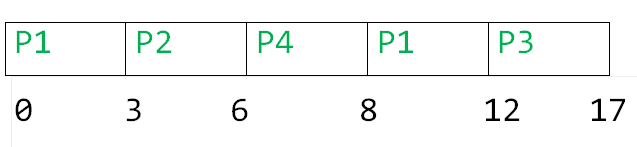# GATE | GATE-CS-2017 (Set 1) | Question 16

Consider the following CPU processes with arrival times (in milliseconds) and length of CPU bursts (in milliseconds) as given below:If the pre-emptive shortest remaining time first scheduling algorithm is used to schedule the processes, then the average waiting time across all processes is _______ milliseconds.

Note: This questions appeared as Numerical Answer Type.
(A) 1
(B) 2
(C) 3
(D) 4

Explanation:Turn Around Time

```P1  = 12-0 = 12
P2 = 6-3 = 3
P3 = 17-5 = 12
P4 = 8 - 6 = 2
```

Waiting Time

```P1  = 12-7 = 5
P2 = 3-3 = 0
P3 = 12-5 = 7
P4 = 2 - 2 = 0
```

Average Waiting time = (7+0+5+0)/4 = 3.0

Therefore, option C is correct

Alternate Solution

Given, with arrival time and burst time:Using (preemptive) shortest remaining time first algorithm, gantt chart is:Therefore,
Average waiting time = ( 5 + 0 + 7 + 0 ) / 4 = 12 / 4 = 3

This explanation has been contributed by Mithlesh Upadhyay.

My Personal Notes arrow_drop_up
Article Tags :

Be the First to upvote.

Please write to us at contribute@geeksforgeeks.org to report any issue with the above content.